# DS博客作业03--树

## 0.PTA得分截图# 1.本周学习总结（5分）

## 1.1 二叉树结构

### 1.1.1 二叉树的2种存储结构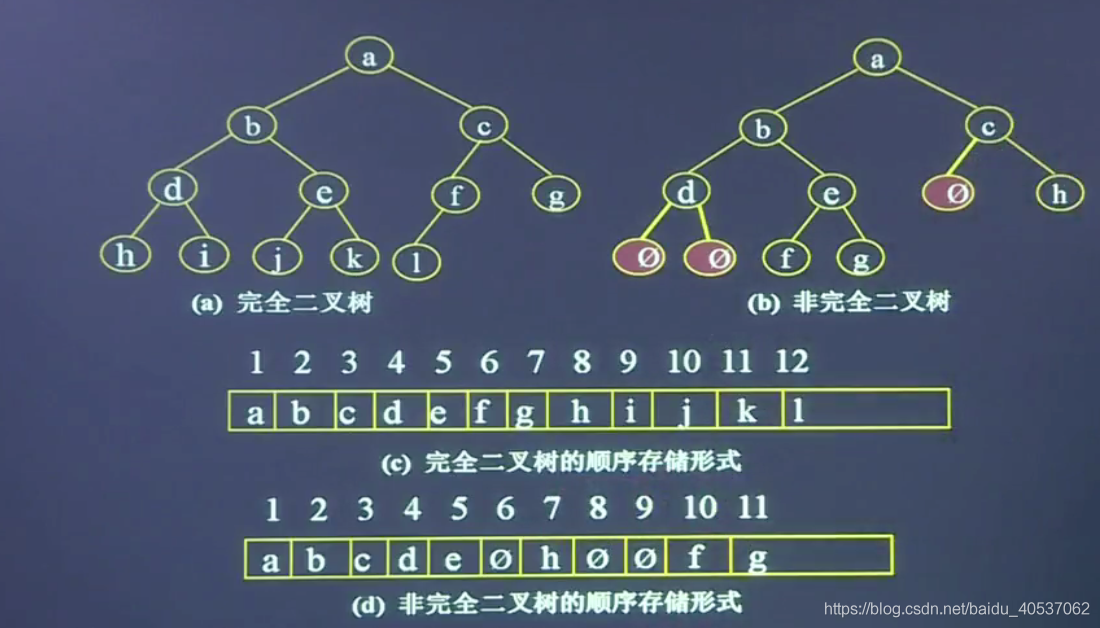#### 顺序存储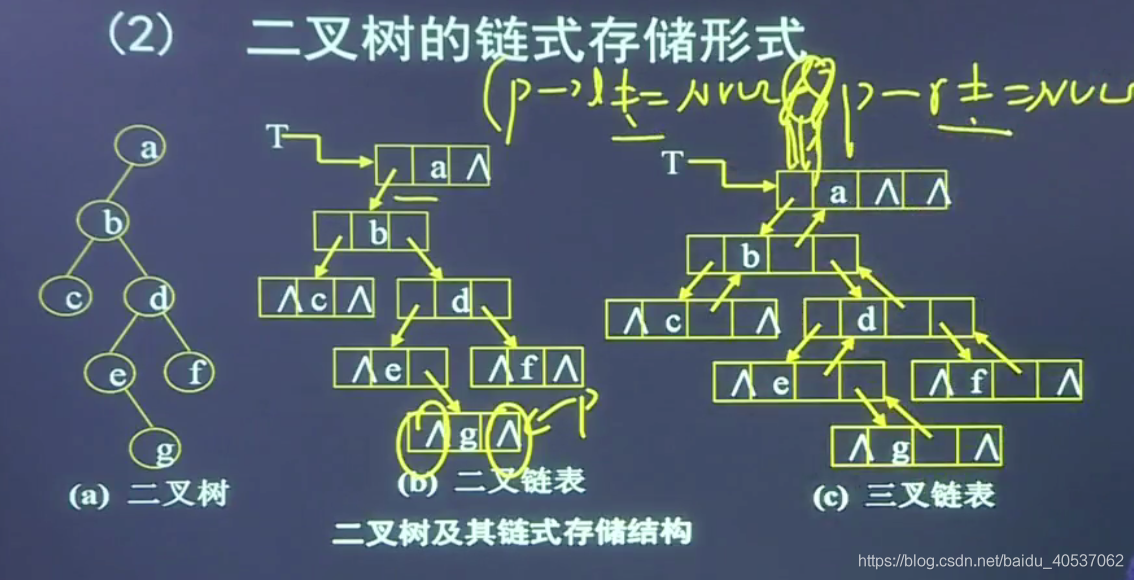### 1.1.2 二叉树的构造

#### 1）先序

BTree CreateBT(string str,int&i)
{
if(i>=len-1)
return NULL;
if(str[i]=='#')
return NULL;

BTree bt=new BTnode;
bt->data=str[i];
bt->lchild=CreateBT(str,++i);
bt->rchild=CreateBT(str,++i);
}


#### 2）中序

BTree CreateBT(string str,int&i)
{
if(i>=len-1)
return NULL;
if(str[i]=='#')
return NULL;
BTree bt=new BTnode;
bt->lchild=CreateBT(str,++i);
bt->data=str[i];
bt->rchild=CreateBT(str,++i);
}


#### 3）后序

BTree CreateBT(string str,int&i)
{
if(i>=len-1)
return NULL;
if(str[i]=='#')
return NULL;
BTree bt=new BTnode;
bt->lchild=CreateBT(str,++i);
bt->rchild=CreateBT(str,++i);
bt->data=str[i];
}


### 1.1.3 二叉树的遍历

#### 1）先序遍历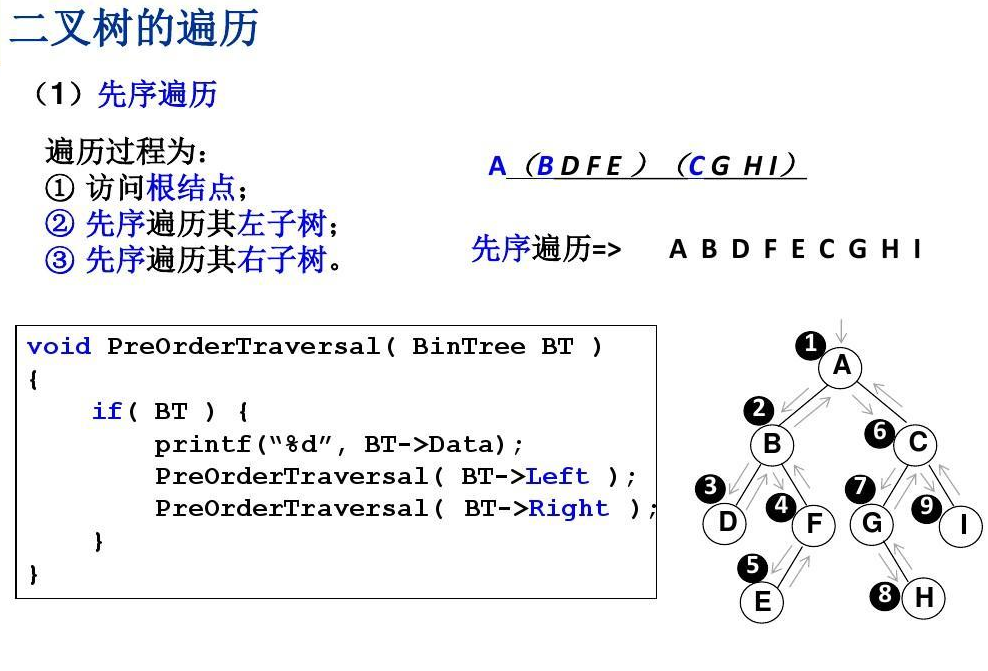void PreOrder(BTree bt)
{
if (bt != NULL)
{
printf("%c", bt->data);//访问根节点
PreOrder(bt->lchild);//先序遍历左子树
PreOrder(bt->rchild);//先序遍历右子树
}
}


#### 2）中序遍历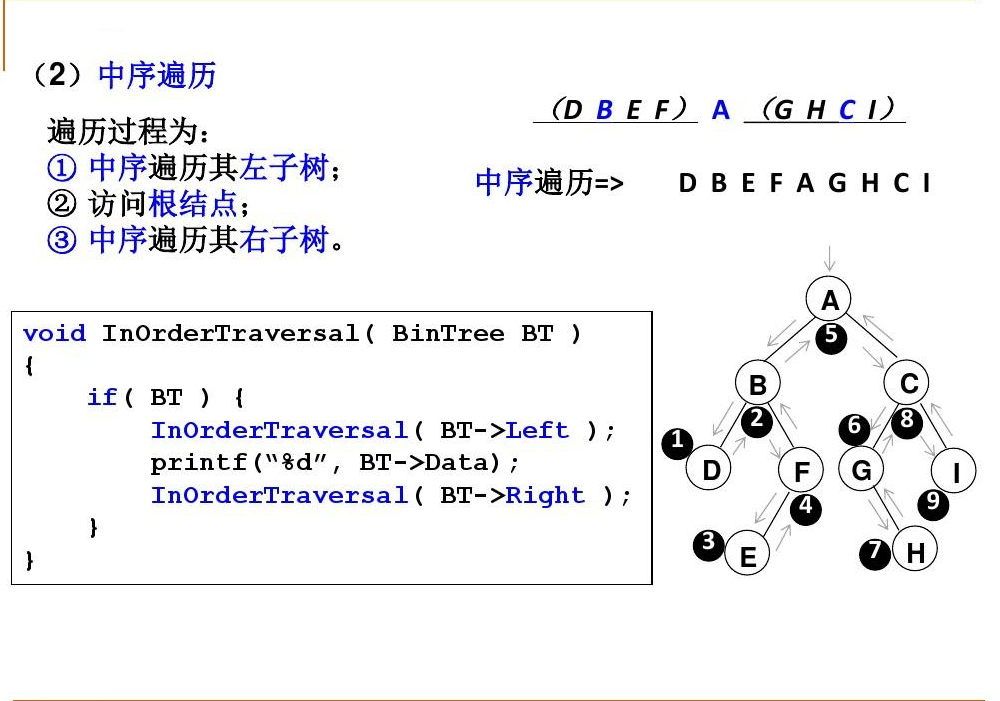void InOrder(BTree bt)
{
if (bt != NULL)
{
InOrder(bt->lchild);//中序遍历左子树
printf("%c", bt->data);//访问根节点
InOrder(bt->rchild);//中序遍历右子树
}
}


#### 3）后序遍历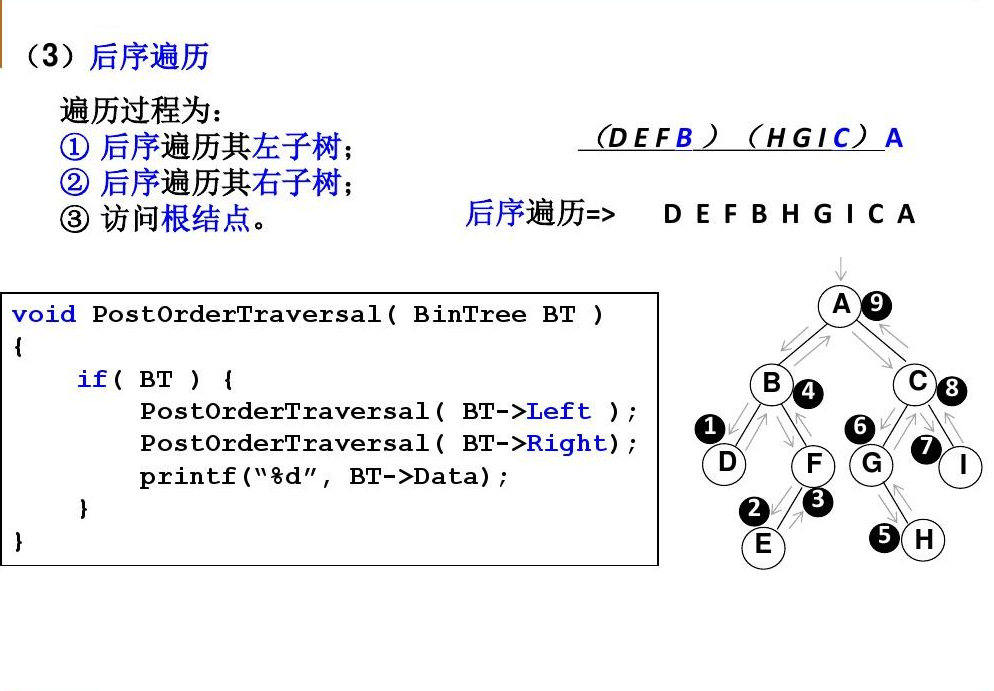void PostOrder(BTree bt)
{
if (bt != NULL)
{
PostOrder(bt->lchild);//遍历左子树
PostOrder(bt->rchild);//遍历右子树
printf("%c", bt->data);//访问根节点
}
}


#### 4）层次遍历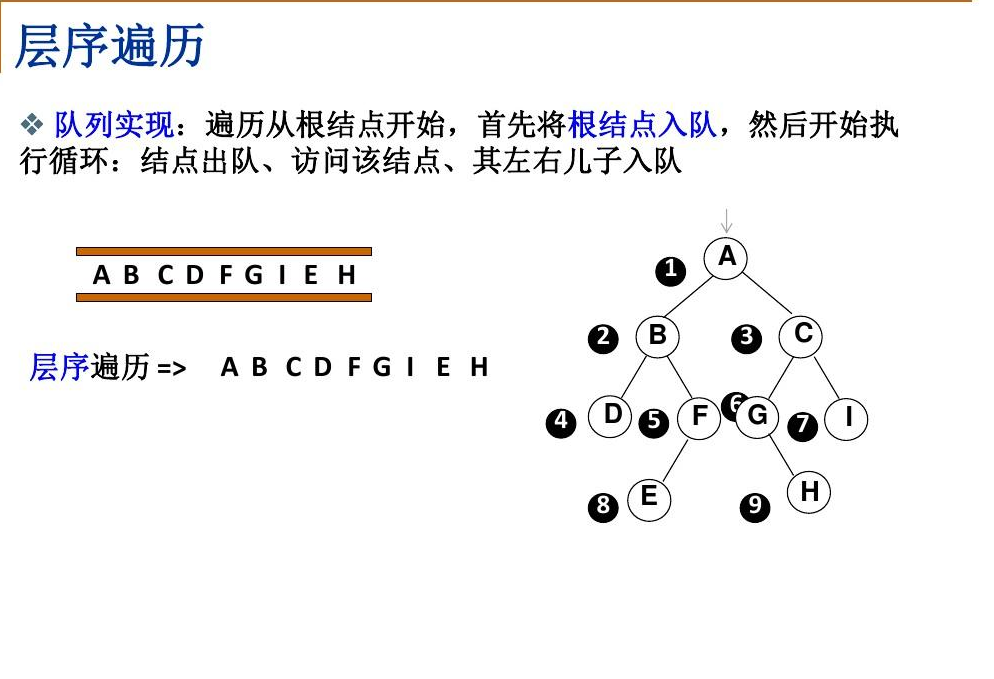void LevelOrder(BinTree bt)//层次遍历
{
queue<BinTree>q;//初始化队列，元素为树节点
BinTree p;
int flag = 0;
if (bt == NULL)//如果树空，输出NULL
{
cout << "NULL";
return;
}
else q.push(bt);//如果树不空，根节点入队

while (!q.empty())//当队列不空
{
p = q.front();//出队访问
q.pop();
if (flag == 0)
{
cout << p->Data;
flag = 1;
}
else
cout << " " << p->Data;

if (p->Left != NULL)//如果该结点有左孩子，入队
q.push(p->Left);
if (p->Right != NULL)//如果该结点有右孩子，入队
q.push(p->Right);
}

}


### 1.1.4 线索二叉树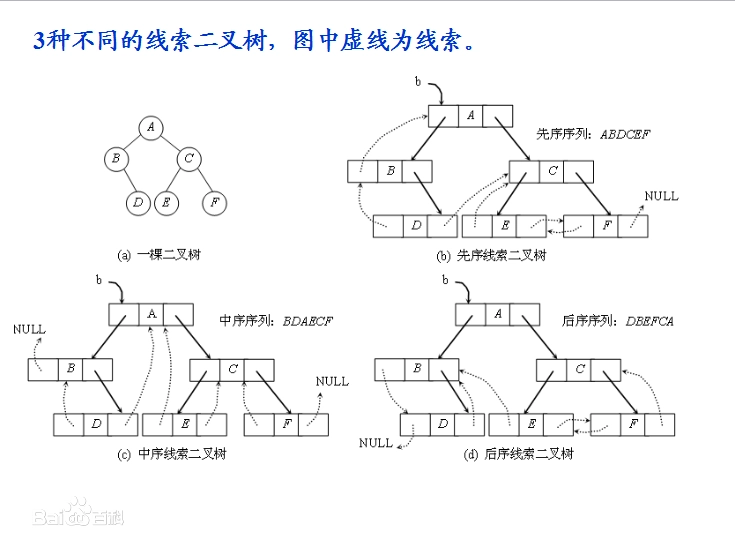### 1.1.5 二叉树的应用--表达式树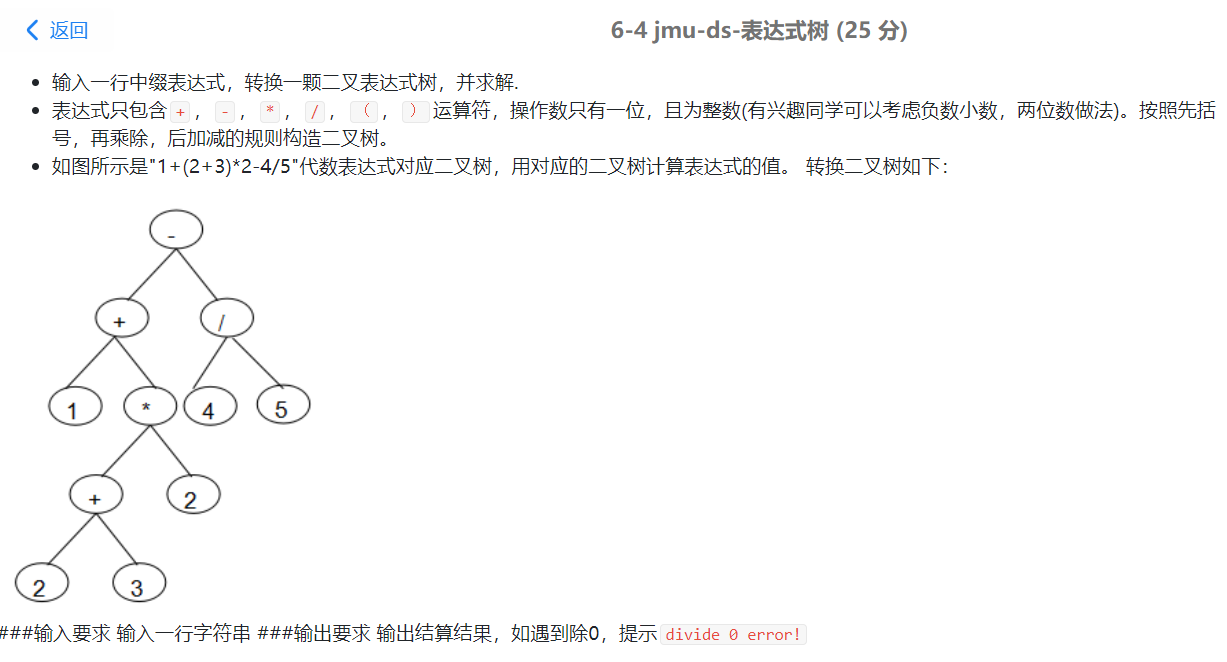void InitExpTree(BTree& T, string str) //建表达式的二叉树
{
stack <BTree> num;//存放数字
stack<char> op;//存放运算符
op.push('#');//必须将#进哦op栈
int i = 0;
while (str[i])
{
if (!In(str[i]))//数字
{
T = new BiTNode;
T->data = str[i++];
T->lchild = T->rchild = NULL;//将左右孩子置空
num.push(T);
}
else//运算符
{
switch (Precede(op.top(), str[i]))
{
case'<':op.push(str[i]); i++; break;//运算符比op栈顶低，入op栈
case'=':op.pop(); i++; break;//运算符与op栈顶相等，op栈顶出栈
case'>':T = new BiTNode;//运算符比op栈顶高，新建结点T
T->data = op.top();//取op栈顶运算符
T->rchild = num.top();//数字栈取栈顶，并出栈
num.pop();
T->lchild = num.top();
num.pop();
num.push(T);//将新建结点入栈
op.pop();//op栈出栈
break;
}
}
}
while (op.top() != '#')
{
T = new BiTNode;
T->data = op.top();
op.pop();
T->rchild = num.top();
num.pop();
T->lchild = num.top();
num.pop();
num.push(T);
}
}

double EvaluateExTree(BTree T)//计算表达式树
{
double a, b;
if (T)
{
if (!T->lchild && !T->rchild)
return T->data-'0';//最终结果
a = EvaluateExTree(T->lchild);
b = EvaluateExTree(T->rchild);
switch (T->data)
{
case'+':return a + b; break;
case'-':return a - b; break;
case'*':return a * b; break;
case'/':
if (b == 0)
{
cout << "divide 0 error!" << endl;
exit(0);
}
return a / b; break;
}
}
}


## 1.2 多叉树结构

### 1.2.1 多叉树结构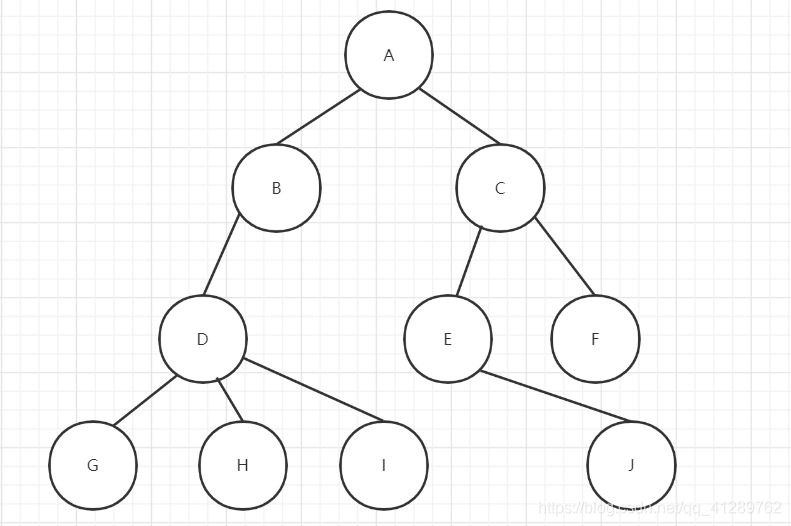#### 孩子兄弟表示法

typedef struct CSNode
{
ElemType data;    //数据域
struct CSNode *firstchild;    //指向对应长子结点的指针域
struct CSNode *rightsib;    //指向对应右兄弟结点的指针域
}CSNode,*CSTree;


## 1.3 哈夫曼树

### 1.3.1 哈夫曼树定义

【路径】树中一个结点到另一个结点之间的分支构成这两个结点之间的路径
【路径长度】路径上的分枝数目称作路径长度
【树的路径长度】从树根到每一个结点的路径长度之和
【权值】指重要程度，在这里指出现的频率。
【结点的带权路径长度WPL】在一棵树中，如果其结点上附带有一个权值，通常把该结点的路径长度与该结点上的权值之积称为该结点的带权路径长度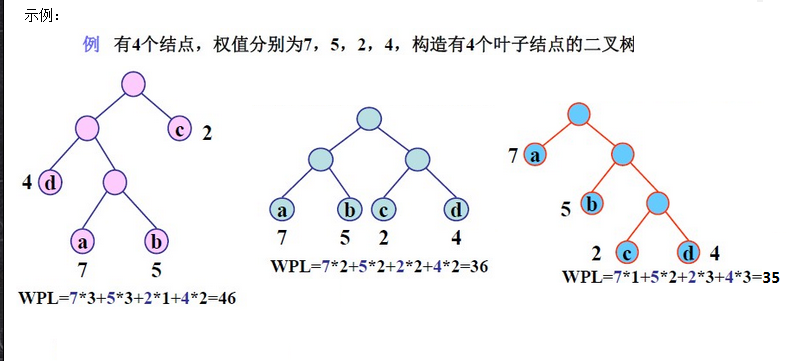### 1.3.2 哈夫曼树的结构体

typedef struct
{	char data;		//节点值
float weight;	//权重
int parent;		//双亲节点
int lchild;		//左孩子节点
int rchild;		//右孩子节点
} HTNode;


### 1.3.2 哈夫曼树构建及哈夫曼编码

#### 哈夫曼树构建

1）8个结点的权值大小如下：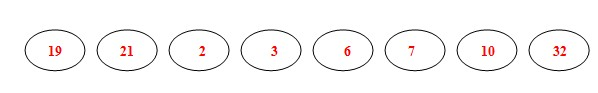2）从19，21，2，3，6，7，10，32中选择两个权小结点。选中2，3。同时算出这两个结点的和5。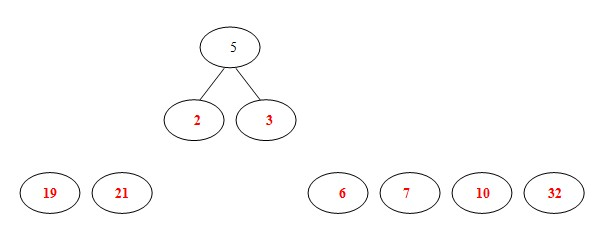3）从19，21，6，7，10，32，5中选出两个权小结点。选中5，6。同时计算出它们的和11。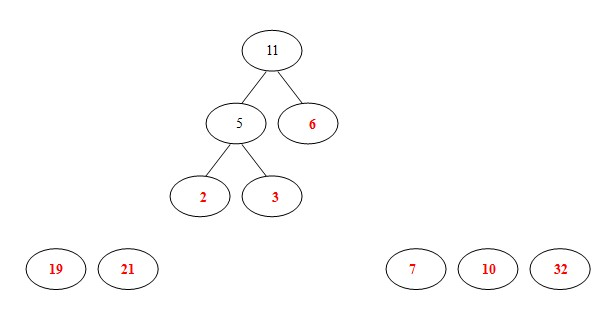4）从19，21，7，10，32，11中选出两个权小结点。选中7，10。同时计算出它们的和17。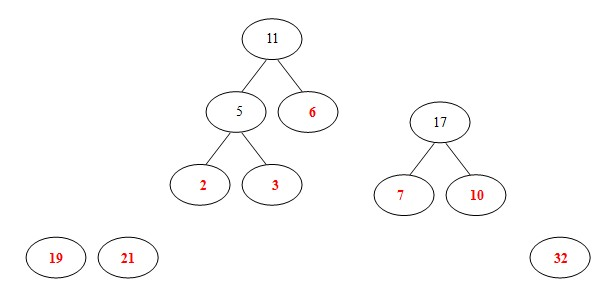5）从19，21，32，11，17中选出两个权小结点。选中11，17。同时计算出它们的和28。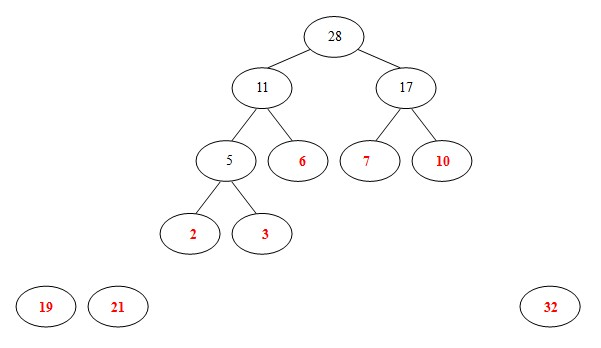6）从19，21，32，28中选出两个权小结点。选中19，21。同时计算出它们的和40。另起一颗二叉树。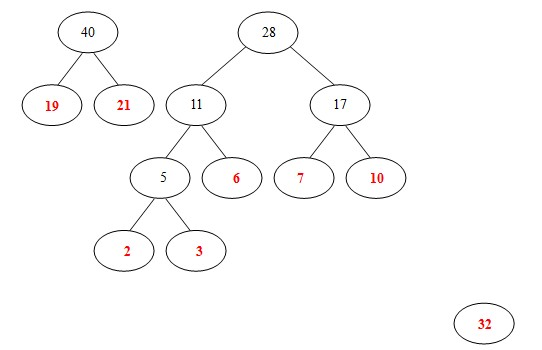7）从32，28， 40中选出两个权小结点。选中28，32。同时计算出它们的和60。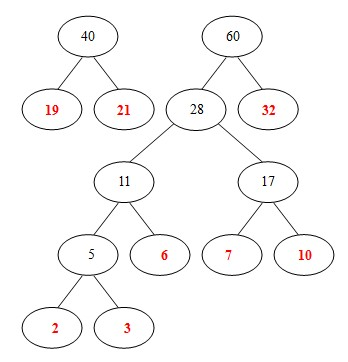8）从 40， 60中选出两个权小结点。选中40，60。同时计算出它们的和100。 好了，此时哈夫曼树已经构建好了。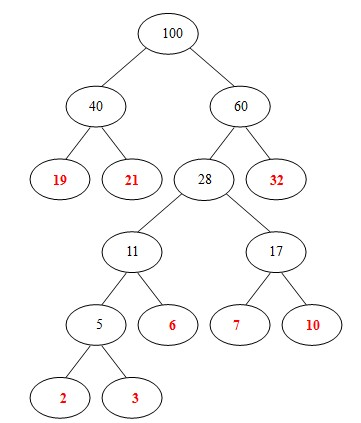#### 哈夫曼编码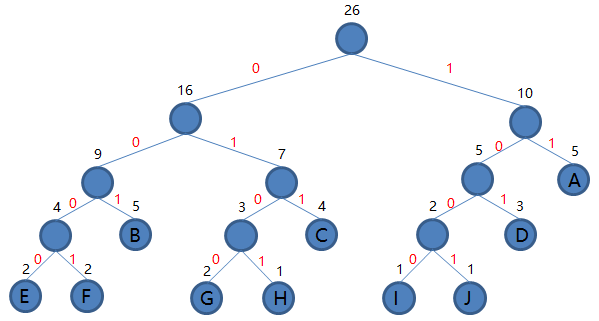## 1.4 并查集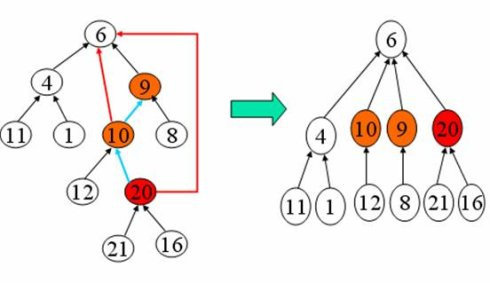#### 1.4.2并查集的结构体、查找、合并实现

1）并查集的结构体

typedef struct node
{      int data;		//结点对应人的编号
int rank;  //结点秩：子树的高度，合并用
int parent;		//结点对应双亲下标
} UFSTree;		//并查集树的结点类型


2）初始化并查集

void MAKE_SET(UFSTree t[]，int n)  //初始化并查集树
{      int i;
for (i=1;i<=n;i++)
{	t[i].data=i;		//数据为该人的编号
t[i].rank=0;		//秩初始化为0
t[i].parent=i;		//双亲初始化指向自已
}
}


3）并查集的查找

int FIND_SET(UFSTree t[]，int x)    //在x所在子树中查找集合编号
{      if (x!=t[x].parent)		                    //双亲不是自已
return(FIND_SET(t，t[x].parent));   //递归在双亲中找x
else
return(x);			      //双亲是自已，返回x
}


4）并查集的合并

void UNION(UFSTree t[]，int x，int y)       //将x和y所在的子树合并
{        x=FIND_SET(t，x);	        //查找x所在分离集合树的编号
y=FIND_SET(t，y);	        //查找y所在分离集合树的编号
if (t[x].rank>t[y].rank)	        //y结点的秩小于x结点的秩
t[y].parent=x;		        //将y连到x结点上，x作为y的双亲结点
else			        //y结点的秩大于等于x结点的秩
{	    t[x].parent=y;		        //将x连到y结点上，y作为x的双亲结点
if (t[x].rank==t[y].rank)      //x和y结点的秩相同
t[y].rank++;	        //y结点的秩增1
}
}


# 2.PTA实验作业（4分）

## 2.1 二叉树叶子结点带权路径长度和

### 2.1.1 解题思路及伪代码

BTree CreatTree(string str,int i)
{
定义树的结构变量bt
当i>len-1或str[i]='#'
返回NULL
申请结点BTNode
将str[i]的值赋给bt->data
递归调用函数CreatTree构建左右孩子
返回bt
}
void GetWPL(BTree bt,int h,int &wpl)
{
判断树是否空，如果树为空，返回NULL
如果左右孩子均不为空
wpl+=bt->data-'0'乘以所在层数
递归调用函数GetWpl，其中的h+1，得到所在层数
}


## 2.2 目录树

### 2.2.1 解题思路及伪代码

void CreatTree(Tree& bt, string str, int i)//建树，
{
新建结点temp，ptr；初始化结点；
切割字符串；新节点name为该段字符；
if 该段字符串为目录，isfile改为false；
if (temp为文件)
InitFile(temp, bt);//插入文件
else //temp为目录
InitList(temp, bt);//插入目录
CreatTree(temp, str, i);
}

void InitList(Tree& temp, Tree& bt)//插入目录
{
定义结构体指针btr来遍历二叉树bt；
btr = bt->child;//btr先指向bt的孩子；

/*先对第一个兄弟结点进行判断*/
if (没有第一个孩子|| btr为文件 || 第一个孩子字典序大于该结点)//可插入
进行插入temp->brother = btr;bt->child = temp;//修改孩子指针
else if (二者相等)
直接使temp指向btr；
else //查找兄弟节点
while (btr->brother != NULL)
if (兄弟节点为文件 || 兄弟节点字典序大于该节点)
找到可插入位置，break；
else if (二者相等)
直接使temp指向btr->brother;break;
else
btr = btr->brother;//遍历下一兄弟结点；
end if
end while
if (btr->brother为空 || btr->brother->name != temp->name)
进行插入操作:temp->brother = btr->brother;btr->brother = temp;
end if
end if
}

void InitFile(Tree& temp, Tree& bt)//对文件temp找一个可插入位置
{
定义结构体指针btr来遍历二叉树bt；
btr = bt->child;//btr先指向bt的孩子；

if (第一个孩子为空 || btr为文件 && 结点字典序大于等于该节点)
进行插入，修改bt的孩子指针；
else //判断兄弟结点
while (btr->brother != NULL)
if (btr->brother为文件 && 兄弟节点字典序大于该节点)
找到可插入位置，break;
else
btr = btr->brother;//遍历下一个兄弟结点
end if
end while
对temp进行插入操作:temp->brother = btr->brother;btr->brother = temp;
end if
}


### 2.2.2 总结解题所用的知识点

1）利用孩子兄弟链结构进行存储;
2）结构体重利用flag队结点进行其实目录还是文件的判断;
3)比较插入结点优先级（因为可能需要改变结点位置）；
4)先序，后序遍历方式；
5)使用递归函数来计算高度。

# 3.阅读代码（0--1分）

## 3.3 分析该题目解题优势及难点。

posted on 2021-05-01 01:33  姚庆荣  阅读(31)  评论(0编辑  收藏  举报